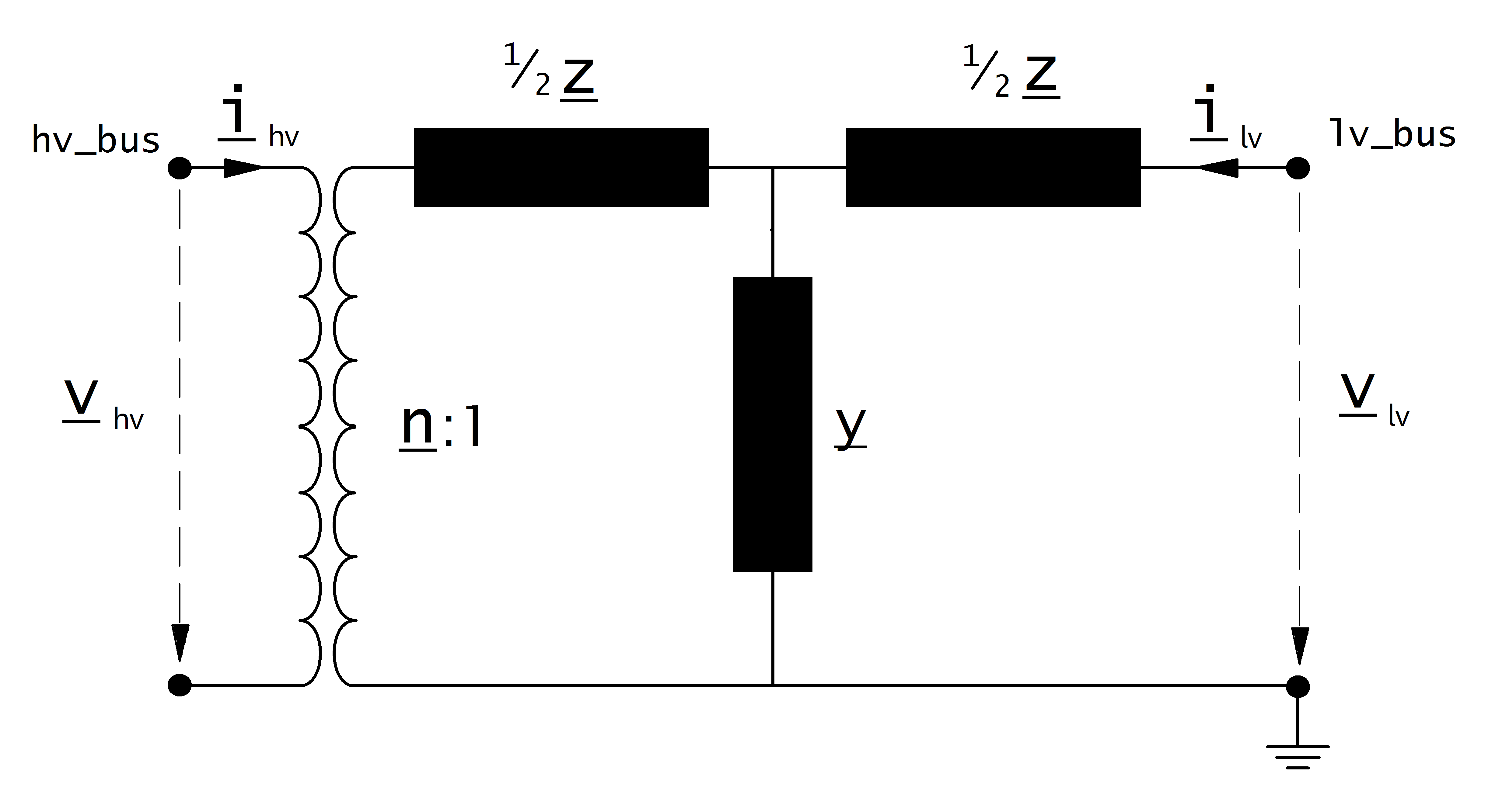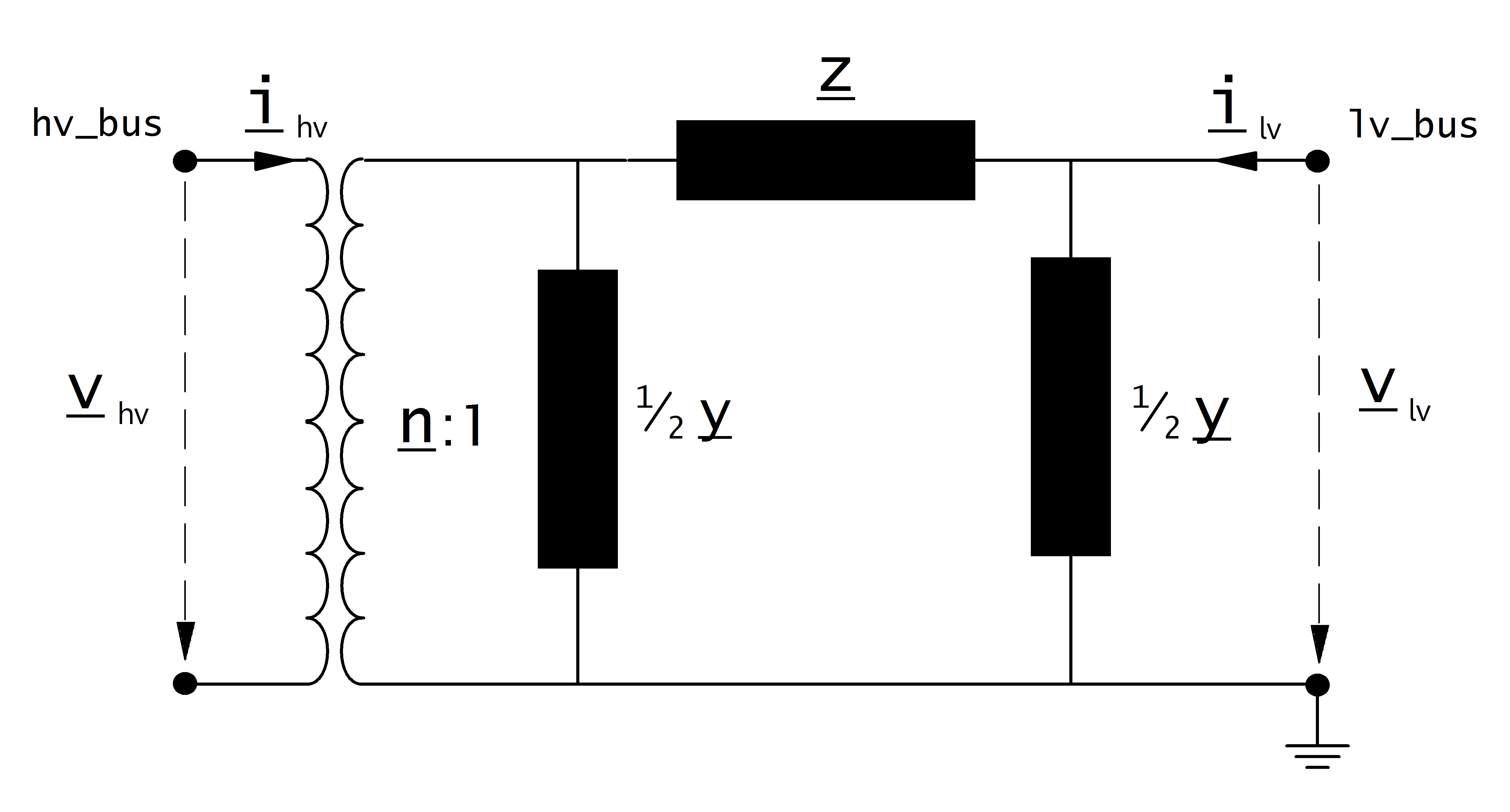# Transformer¶

## Create Function¶

Transformers can be either created from the standard type library (create_transformer) or with custom values (create_transformer_from_parameters).

pandapower.create_transformer(net, hv_bus, lv_bus, std_type, name=None, tap_pos=nan, in_service=True, index=None, max_loading_percent=nan, parallel=1, df=1.0)

Creates a two-winding transformer in table net[“trafo”]. The trafo parameters are defined through the standard type library.

INPUT:

net - The net within this transformer should be created

hv_bus (int) - The bus on the high-voltage side on which the transformer will be connected to

lv_bus (int) - The bus on the low-voltage side on which the transformer will be connected to

std_type - The used standard type from the standard type library

OPTIONAL:

name (string, None) - A custom name for this transformer

tap_pos (int, nan) - current tap position of the transformer. Defaults to the medium position (tap_neutral)

in_service (boolean, True) - True for in_service or False for out of service

index (int, None) - Force a specified ID if it is available. If None, the index one higher than the highest already existing index is selected.

parallel (integer) - number of parallel transformers

df (float) - derating factor: maximal current of transformer in relation to nominal current of transformer (from 0 to 1)

OUTPUT:

index (int) - The unique ID of the created transformer

EXAMPLE:

create_transformer(net, hv_bus = 0, lv_bus = 1, name = “trafo1”, std_type = “0.4 MVA 10/0.4 kV”)

pandapower.create_transformer_from_parameters(net, hv_bus, lv_bus, sn_mva, vn_hv_kv, vn_lv_kv, vkr_percent, vk_percent, pfe_kw, i0_percent, shift_degree=0, tap_side=None, tap_neutral=nan, tap_max=nan, tap_min=nan, tap_step_percent=nan, tap_step_degree=nan, tap_pos=nan, tap_phase_shifter=False, in_service=True, name=None, index=None, max_loading_percent=nan, parallel=1, df=1.0, **kwargs)

Creates a two-winding transformer in table net[“trafo”]. The trafo parameters are defined through the standard type library.

INPUT:

net - The net within this transformer should be created

hv_bus (int) - The bus on the high-voltage side on which the transformer will be connected to

lv_bus (int) - The bus on the low-voltage side on which the transformer will be connected to

sn_mva (float) - rated apparent power

vn_hv_kv (float) - rated voltage on high voltage side

vn_lv_kv (float) - rated voltage on low voltage side

vkr_percent (float) - real part of relative short-circuit voltage

vk_percent (float) - relative short-circuit voltage

pfe_kw (float) - iron losses in kW

i0_percent (float) - open loop losses in percent of rated current

OPTIONAL:

in_service (boolean) - True for in_service or False for out of service

parallel (integer) - number of parallel transformers

name (string) - A custom name for this transformer

shift_degree (float) - Angle shift over the transformer*

tap_side (string) - position of tap changer (“hv”, “lv”)

tap_pos (int, nan) - current tap position of the transformer. Defaults to the medium position (tap_neutral)

tap_neutral (int, nan) - tap position where the transformer ratio is equal to the ration of the rated voltages

tap_max (int, nan) - maximal allowed tap position

tap_min (int, nan): minimal allowed tap position

tap_step_percent (float) - tap step size for voltage magnitude in percent

tap_step_degree (float) - tap step size for voltage angle in degree*

tap_phase_shifter (bool) - whether the transformer is an ideal phase shifter*

index (int, None) - Force a specified ID if it is available. If None, the index one higher than the highest already existing index is selected.

df (float) - derating factor: maximal current of transformer in relation to nominal current of transformer (from 0 to 1)

** only considered in loadflow if calculate_voltage_angles = True

OUTPUT:

index (int) - The unique ID of the created transformer

EXAMPLE:

create_transformer_from_parameters(net, hv_bus=0, lv_bus=1, name=”trafo1”, sn_mva=40, vn_hv_kv=110, vn_lv_kv=10, vk_percent=10, vkr_percent=0.3, pfe_kw=30, i0_percent=0.1, shift_degree=30)

## Input Parameters¶

net.trafo

 Parameter Datatype Value Range Explanation name string name of the transformer std_type string transformer standard type name hv_bus* integer high voltage bus index of the transformer lv_bus* integer low voltage bus index of the transformer sn_mva* float $$>$$ 0 rated apparent power of the transformer [MVA] vn_hv_kv* float $$>$$ 0 rated voltage at high voltage bus [kV] vn_lv_kv* float $$>$$ 0 rated voltage at low voltage bus [kV] vk_percent* float $$>$$ 0 short circuit voltage [%] vkr_percent* float $$\geq$$ 0 real component of short circuit voltage [%] pfe_kw* float $$\geq$$ 0 iron losses [kW] i0_percent* float $$\geq$$ 0 open loop losses in [%] shift_degree* float transformer phase shift angle tap_side string “hv”, “lv” defines if tap changer is at the high- or low voltage side tap_neutral integer rated tap position tap_min integer minimum tap position tap_max integer maximum tap position tap_step_percent float $$>$$ 0 tap step size for voltage magnitude [%] tap_step_degree float $$\geq$$ 0 tap step size for voltage angle tap_pos integer current position of tap changer tap_phase_shifter bool defines whether the transformer is an ideal phase shifter parallel int $$>$$ 0 number of parallel transformers max_loading_percent** float $$>$$ 0 Maximum loading of the transformer with respect to sn_mva and its corresponding current at 1.0 p.u. df float 1 $$\geq$$ df :math:>0 derating factor: maximal current of transformer in relation to nominal current of transformer (from 0 to 1) in_service* boolean True / False specifies if the transformer is in service.

*necessary for executing a power flow calculation
**optimal power flow parameter

Note

## Electric Model¶

The equivalent circuit used for the transformer can be set in the power flow with the parameter “trafo_model”.

trafo_model=’t’:trafo_model=’pi’:### Transformer Ratio¶

The magnitude of the transformer ratio is given as:

\begin{align*} n &= \frac{V_{ref, HV, transformer}}{V_{ref, LV, transformer}} \cdot \frac{V_{ref, LV bus}}{V_{ref, HV bus}} \end{align*}

The reference voltages of the high- and low voltage buses are taken from the net.bus table. The reference voltage of the transformer is taken directly from the transformer table:

\begin{align*} V_{ref, HV, transformer} &= vn\_hv\_kv \\ V_{ref, LV, transformer} &= vn\_lv\_kv \end{align*}

If the power flow is run with voltage_angles=True, the complex ratio is given as:

\begin{align*} \underline{n} &= n \cdot e^{j \cdot \theta \cdot \frac{\pi}{180}} \\ \theta &= shift\_degree \end{align*}

Otherwise, the ratio does not include a phase shift:

\begin{align*} \underline{n} &= n \end{align*}

### Impedance Values¶

The short-circuit impedance is calculated as:

\begin{align*} z_k &= \frac{vk\_percent}{100} \cdot \frac{1000}{sn\_mva} \\ r_k &= \frac{vkr\_percent}{100} \cdot \frac{1000}{sn\_mva} \\ x_k &= \sqrt{z^2 - r^2} \\ \underline{z}_k &= r_k + j \cdot x_k \end{align*}

The magnetising admittance is calculated as:

\begin{align*} y_m &= \frac{i0\_percent}{100} \\ g_m &= \frac{pfe\_mw}{sn\_mva \cdot 1000} \cdot \frac{1000}{sn\_mva} \\ b_m &= \sqrt{y_m^2 - g_m^2} \\ \underline{y_m} &= g_m - j \cdot b_m \end{align*}

The values calculated in that way are relative to the rated values of the transformer. To transform them into the per unit system, they have to be converted to the rated values of the network:

\begin{align*} Z_{N} &= \frac{V_{N}^2}{S_{N}} \\ Z_{ref, trafo} &= \frac{vn\_lv\_kv^2 \cdot 1000}{sn\_mva} \\ \underline{z} &= \underline{z}_k \cdot \frac{Z_{ref, trafo}}{Z_{N}} \\ \underline{y} &= \underline{y}_m \cdot \frac{Z_{N}}{Z_{ref, trafo}} \\ \end{align*}

Where the reference voltage $$V_{N}$$ is the nominal voltage at the low voltage side of the transformer and the rated apparent power $$S_{N}$$ is defined system wide in the net object (see Unit Systems and Conventions).

### Tap Changer¶

Longitudinal regulator

A longitudinal regulator can be modeled by setting tap_phase_shifter to False and defining the tap changer voltage step with tap_step_percent.

The reference voltage is then multiplied with the tap factor:

\begin{align*} n_{tap} = 1 + (tap\_pos - tap\_neutral) \cdot \frac{tap\_st\_percent}{100} \end{align*}

On which side the reference voltage is adapted depends on the $$tap\_side$$ variable:

 tap_side=”hv” tap_side=”lv” $$V_{n, HV, transformer}$$ $$vnh\_kv \cdot n_{tap}$$ $$vnh\_kv$$ $$V_{n, LV, transformer}$$ $$vnl\_kv$$ $$vnl\_kv \cdot n_{tap}$$

Note

The variables tap_min and tap_max are not considered in the power flow. The user is responsible to ensure that tap_min < tap_pos < tap_max!

Cross regulator

In addition to tap_step_percent a value for tap_step_degree can be defined to model an angle shift for each tap, resulting in a cross regulator that affects the magnitude as well as the angle of the transformer ratio.

Ideal phase shifter

If tap_phase_shifter is set to True, the tap changer is modeled as an ideal phase shifter, meaning that a constant angle shift is added with each tap step:

\begin{align*} \underline{n} &= n \cdot e^{j \cdot (\theta + \theta_{tp}) \cdot \frac{\pi}{180}} \\ \theta &= shift\_degree \end{align*}

The angle shift can be directly defined in tap_step_degree, in which case:

\begin{align*} \theta_{tp} = tap\_st\_degree \cdot (tap\_pos - tap\_neutral) \end{align*}

or it can be given as a constant voltage step in tap_step_percent, in which case the angle is calculated as:

\begin{align*} \theta_{tp} = 2 \cdot arcsin(\frac{1}{2} \cdot \frac{tap\_st\_percent}{100}) \cdot (tap\_pos - tap\_neutral) \end{align*}

If both values are given for an ideal phase shift transformer, the power flow will raise an error.

## Result Parameters¶

net.res_trafo

 Parameter Datatype Explanation p_hv_mw float active power flow at the high voltage transformer bus [MW] q_hv_mvar float reactive power flow at the high voltage transformer bus [MVar] p_lv_mw float active power flow at the low voltage transformer bus [MW] q_lv_mvar float reactive power flow at the low voltage transformer bus [MVar] pl_mw float active power losses of the transformer [MW] ql_mvar float reactive power consumption of the transformer [Mvar] i_hv_ka float current at the high voltage side of the transformer [kA] i_lv_ka float current at the low voltage side of the transformer [kA] vm_hv_pu float voltage magnitude at the low voltage bus [pu] vm_lv_pu float voltage magnitude at the high voltage bus [pu] va_hv_degree float voltage angle at the low voltage bus [degrees] va_lv_degree float voltage angle at the high voltage bus [degrees] loading_percent float load utilization relative to rated power [%]
\begin{align*} p\_hv\_mw &= Re(\underline{v}_{hv} \cdot \underline{i}^*_{hv}) \\ q\_hv\_mvar &= Im(\underline{v}_{hv} \cdot \underline{i}^*_{hv}) \\ p\_lv\_mw &= Re(\underline{v}_{lv} \cdot \underline{i}^*_{lv}) \\ q\_lv\_mvar &= Im(\underline{v}_{lv} \cdot \underline{i}^*_{lv}) \\ pl\_mw &= p\_hv\_mw + p\_lv\_mw \\ ql\_mvar &= q\_hv\_mvar + q\_lv\_mvar \\ i\_hv\_ka &= i_{hv} \\ i\_lv\_ka &= i_{lv} \end{align*}